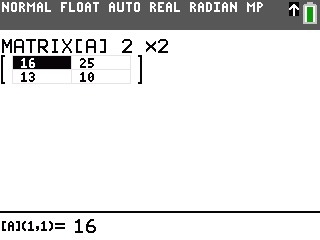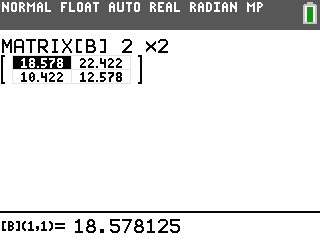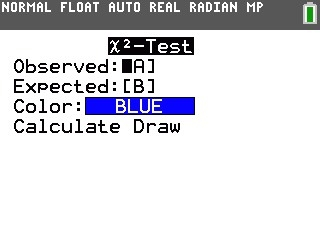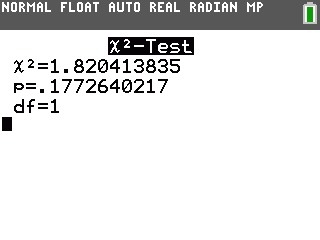# Knowledge Base

## Solution 34872: Performing Chi-Square Computations on the TI-83 Plus and TI-84 Plus Family of Graphing Calculators.

### How do I compute chi-square on the TI-83 Plus and TI-84 Plus family of graphing calculators?

The following example demonstrates how to perform c2 (chi-square) calculations.

Matrices for this example:

Observed Matrix=Expected Matrix=1) Enter the matrix editor, press [2nd][MATRX]
2) Move the cursor to highlight EDIT and select the matrix A.
3) Input the data for the Observed Matrix.
4) The Expected Matrix values will be inputted into Matrix B after you compute the c2 test

Compute the c2 test:

1) Press [STAT] and move cursor to highlight TESTS.
2) Select C:c2-Test.
Please Note: If you did not use the default matrix [A] the matrices will need to be changed, the names can be accessed by pressing [2nd] [MATRX] then pressing the number of the matrix you have stored the O values to.
Additional Note:The Color option only applies to the "Draw" option and is only available on the TI-84 Plus CE and TI-84 Plus C Silver Edition.
3) Once this is done, highlight Calculate to display the calculated results or Draw for the graph.Please see the TI-83 Plus and TI-84 Plus Family guidebooks for additional information.